Lec 25 - Sequences & Series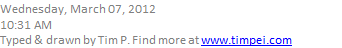Recall: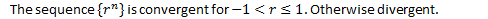Definition

Increasing, decreasing, monotonic, bounded sequence.Aside: Completeness Axiom

If a set of real numbers has an upper bound then there is a least upper bound.Theorem: Monotonic Sequence Theorem

Every bounded, monotonic sequence is convergent.

Proof:

1.By the axiom above, if it is bounded there is a least upper bound.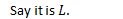1.1.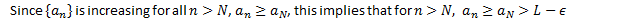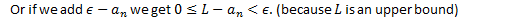1.This implies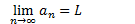Example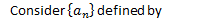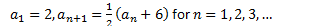Proof:Play with the sequence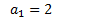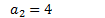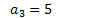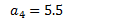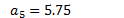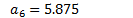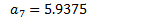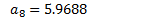1. Increasing: Proof by induction.
1.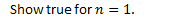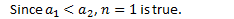1.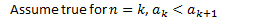1.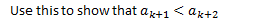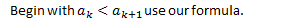1. Bounded: Proof by induction.1.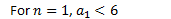1.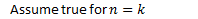1.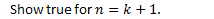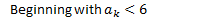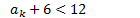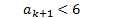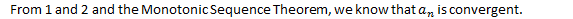1. To find the limit, take the limit of the formula.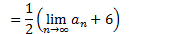Taking the limit,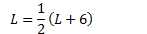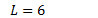Therefore,Series 11.2

Definition

An infinite series is an infinite sum of a sequence,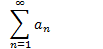DefinitionDefinition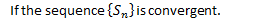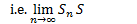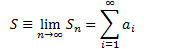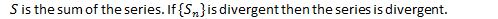Definition

The geometric series is the sum of the geometric sequence.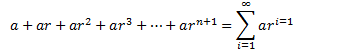Created by Tim Pei with Microsoft OneNote 2010
One place for all your notes and information